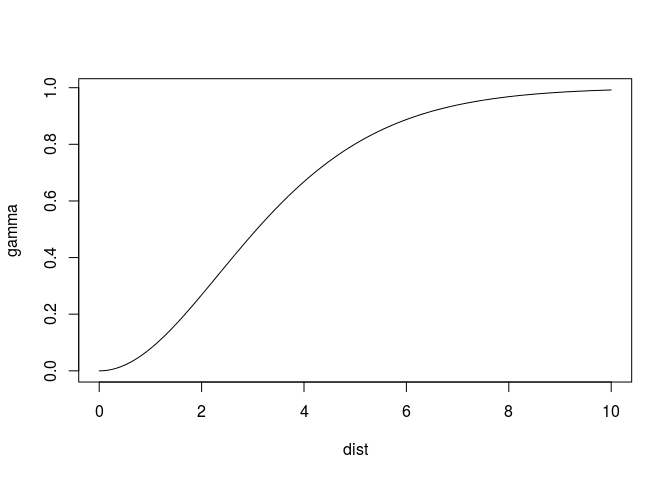view raw Rmd

Fitting variogram functions with R package gstat has become more flexible, and hopefully more user friendly. Up to now, after loading data

``````library(sp)
demo(meuse, ask = FALSE, echo = FALSE) # load meuse data set
``````

users were required to use a sequence like

``````library(gstat)
v = variogram(log(zinc)~1, meuse)
v.fit = fit.variogram(v, vgm(1, "Sph", 900, 1))
v.fit

##   model      psill    range
## 1   Nug 0.05066243   0.0000
## 2   Sph 0.59060780 897.0209
``````

where `fit.variogram` fits variogram parameters of a spherical model (`Sph`) to the sample variogram `v`. The values 1, 900 and 1 were needed as initial values in the weighted non-linear fit (where only the range parameter is non-linear).

This has changed in gstat version 1.2: now, `vgm` can take only a variogram model, as in

``````fit.variogram(v, vgm("Sph"))

##   model      psill    range
## 1   Nug 0.05065971   0.0000
## 2   Sph 0.59060511 897.0011
``````

or even a set of models, in which case the best fitting is returned, as in

``````fit.variogram(v, vgm(c("Exp", "Sph")))

##   model      psill    range
## 1   Nug 0.05065971   0.0000
## 2   Sph 0.59060511 897.0011

fit.variogram(v, vgm(c("Exp", "Mat", "Sph")))

##   model      psill    range
## 1   Nug 0.05065971   0.0000
## 2   Sph 0.59060511 897.0011
``````

where we still see that the sperical model is chosen. If we choose a different sample variogram, where Matern is chosen, as in:

``````v0 = variogram(zinc~1, meuse)
fit.variogram(v0, vgm(c("Exp", "Mat", "Sph")))

##   model    psill    range kappa
## 1   Nug   9486.4   0.0000   0.0
## 2   Mat 163285.3 381.7076   0.5
``````

we see that the kappa value is 0.5, which is a default value that was not fit. We can fit kappa by specifying `fit.kappa = TRUE`, as in

``````options(warn = -1) # don't print warnings
fit.variogram(v0, vgm(c("Exp", "Mat", "Sph")), fit.kappa = TRUE)

##   model    psill    range kappa
## 1   Nug      0.0   0.0000   0.0
## 2   Mat 176455.5 456.4375   0.4
``````

where the best fitting kappa from the range 0.3, 0.4, 0.5,…,5 is chosen. I suppressed warnings here, as around 20 warnings were printed in cases with crazy initial values. This is usual for Matern models: larger kappa values have effective ranges (the distance value at which the model reaches, say, 95% of its sill) much larger than the range parameter, as illustrated by

``````plot(variogramLine(vgm(1, "Mat", 1, kappa = 4), 10), type = 'l')
``````where at distance 1, 0.05 of the sill is reached (and the model, up till there, is nearly linear or parabolic, leading to a singularity during fit). A different parameterisation of the Matern model, given in Michael Stein’s book, is the following

``````plot(variogramLine(vgm(1, "Ste", 1, kappa = 4), 10), type = 'l')
``````This one has the same smoothness, but reaches the sill much closer to the range value. As a consequence it fits easier, that is, without warnings:

``````options(warn = 0) # set back to normal
fit.variogram(v0, vgm(c("Exp", "Ste", "Sph")), fit.kappa = TRUE)

##   model    psill    range kappa
## 1   Nug      0.0   0.0000   0.0
## 2   Ste 176455.4 577.3522   0.4
``````

For those you need a more precise estimate of the optimal kappa value, you can iterate over steps of e.g. 0.01 by

``````fit.variogram(v0, vgm(c("Exp", "Ste", "Sph")), fit.kappa = seq(.3,5,.01))

##   model       psill    range kappa
## 1   Nug    282.7529   0.0000  0.00
## 2   Ste 175030.7434 563.3126  0.41
``````

## How it works

Default initial parameter values are chosen from the sample variogram, where:

• the range parameter is taken as 1/3 of the maximum sample variogram distance,
• the nugget parameter is taken as the mean of the first three sample variogram values, and
• the partial sill is taken as the mean of the last five sample variogram values.
``````vgm("Sph")

##   model psill range
## 1   Nug    NA     0
## 2   Sph    NA    NA
``````

contains `NA` values for the numeric parameters, and under the hood (undocumented)

``````gstat:::vgm_fill_na(vgm("Sph"), v)

##   model     psill    range
## 1   Nug 0.2141508   0.0000
## 2   Sph 0.6200691 514.4008
``````

fills the `NA` values with the initial values.

Providing more than one model to `vgm` returns a list,

``````vgm(c("Sph", "Exp"))

## []
##   model psill range
## 1   Nug    NA     0
## 2   Sph    NA    NA
##
## []
##   model psill range
## 1   Nug    NA     0
## 2   Exp    NA    NA
##
## attr(,"class")
##  "variogramModelList" "list"
``````

which `fit.variogram` iterates over, returning the best fitting model.

## Comparison to automap

Function `automap::autofitVariogram` does a similar job, but includes the computation of the sample variogram from data (which can be controlled by passing parameters to `...`). It takes slightly different defaults for fitting, definitely different defaults when computing the sample variogram, and has options for combining distance bins.